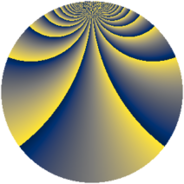# Properties

 Label 2001.2.brLevel $2001$ Weight $2$ Character orbit 2001.br Rep. character $\chi_{2001}(5,\cdot)$ Character field $\Q(\zeta_{154})$ Dimension $14160$ Sturm bound $480$

# Related objects

## Defining parameters

 Level: $$N$$ $$=$$ $$2001 = 3 \cdot 23 \cdot 29$$ Weight: $$k$$ $$=$$ $$2$$ Character orbit: $$[\chi]$$ $$=$$ 2001.br (of order $$154$$ and degree $$60$$) Character conductor: $$\operatorname{cond}(\chi)$$ $$=$$ $$2001$$ Character field: $$\Q(\zeta_{154})$$ Sturm bound: $$480$$

## Dimensions

The following table gives the dimensions of various subspaces of $$M_{2}(2001, [\chi])$$.

Total New Old
Modular forms 14640 14640 0
Cusp forms 14160 14160 0
Eisenstein series 480 480 0

## Trace form

 $$14160q - 63q^{3} + 142q^{4} - 27q^{6} - 110q^{7} - 53q^{9} + O(q^{10})$$ $$14160q - 63q^{3} + 142q^{4} - 27q^{6} - 110q^{7} - 53q^{9} - 154q^{10} - 70q^{13} - 77q^{15} + 150q^{16} - 63q^{18} - 154q^{19} - 77q^{21} - 168q^{24} + 138q^{25} - 105q^{27} - 264q^{28} - 132q^{30} - 126q^{31} - 55q^{33} - 110q^{34} - 15q^{36} - 154q^{37} - 21q^{39} - 154q^{40} - 55q^{42} - 154q^{43} - 140q^{48} - 882q^{49} - 55q^{51} - 126q^{52} - 53q^{54} - 378q^{55} - 66q^{57} - 174q^{58} - 77q^{60} - 154q^{61} - 165q^{63} - 77q^{66} - 66q^{67} - 126q^{69} - 21q^{72} - 126q^{73} - 154q^{76} + 125q^{78} - 154q^{79} - 85q^{81} + 78q^{82} - 77q^{84} - 406q^{85} - 184q^{87} - 440q^{88} - 77q^{90} - 708q^{93} - 86q^{94} + 84q^{96} - 154q^{97} + O(q^{100})$$

## Decomposition of $$S_{2}^{\mathrm{new}}(2001, [\chi])$$ into newform subspaces

The newforms in this space have not yet been added to the LMFDB.